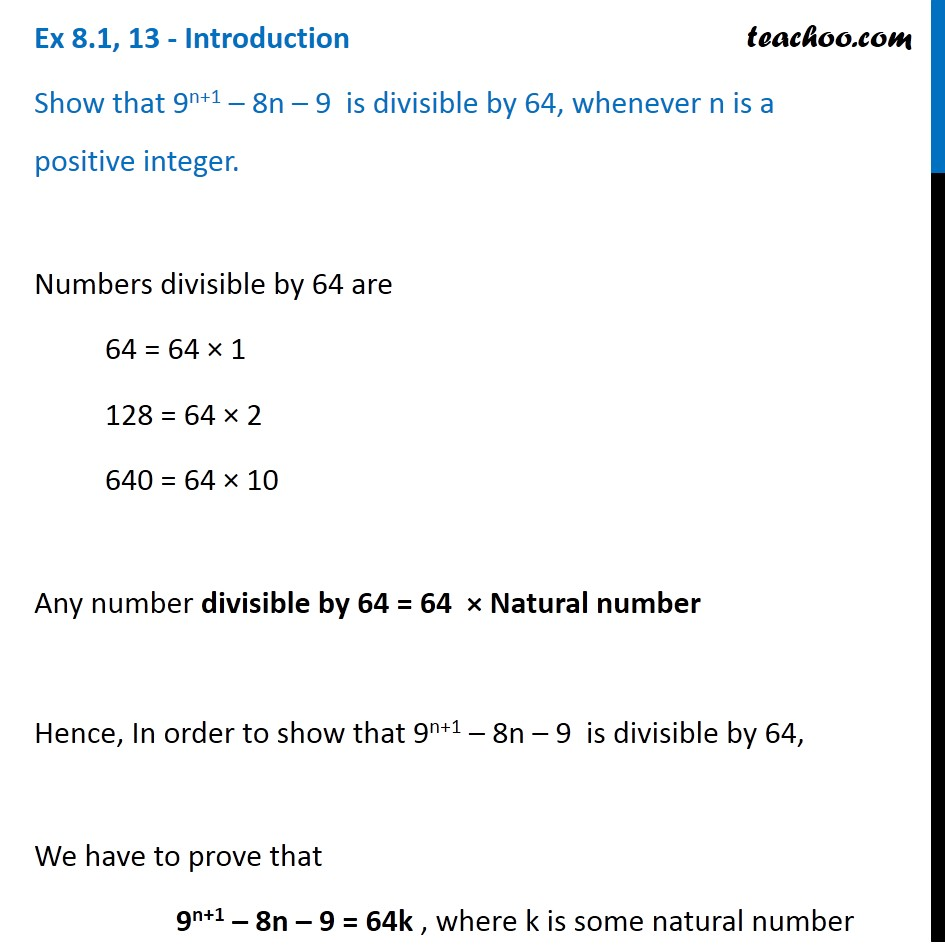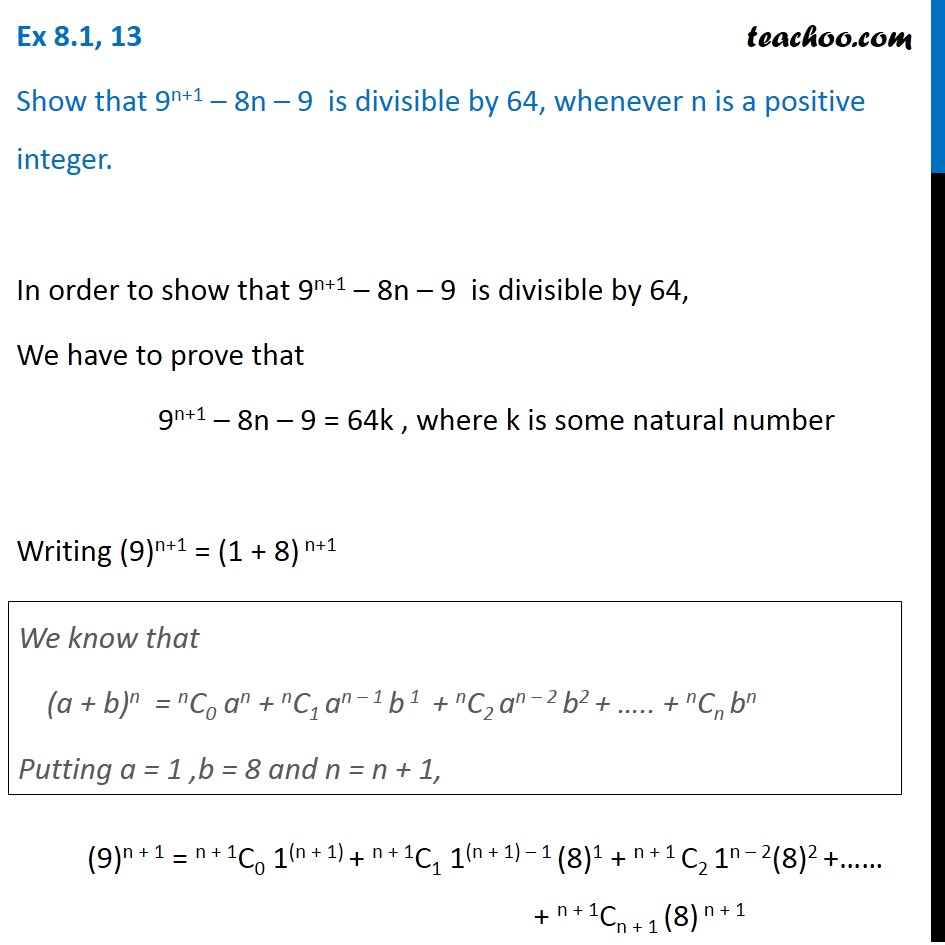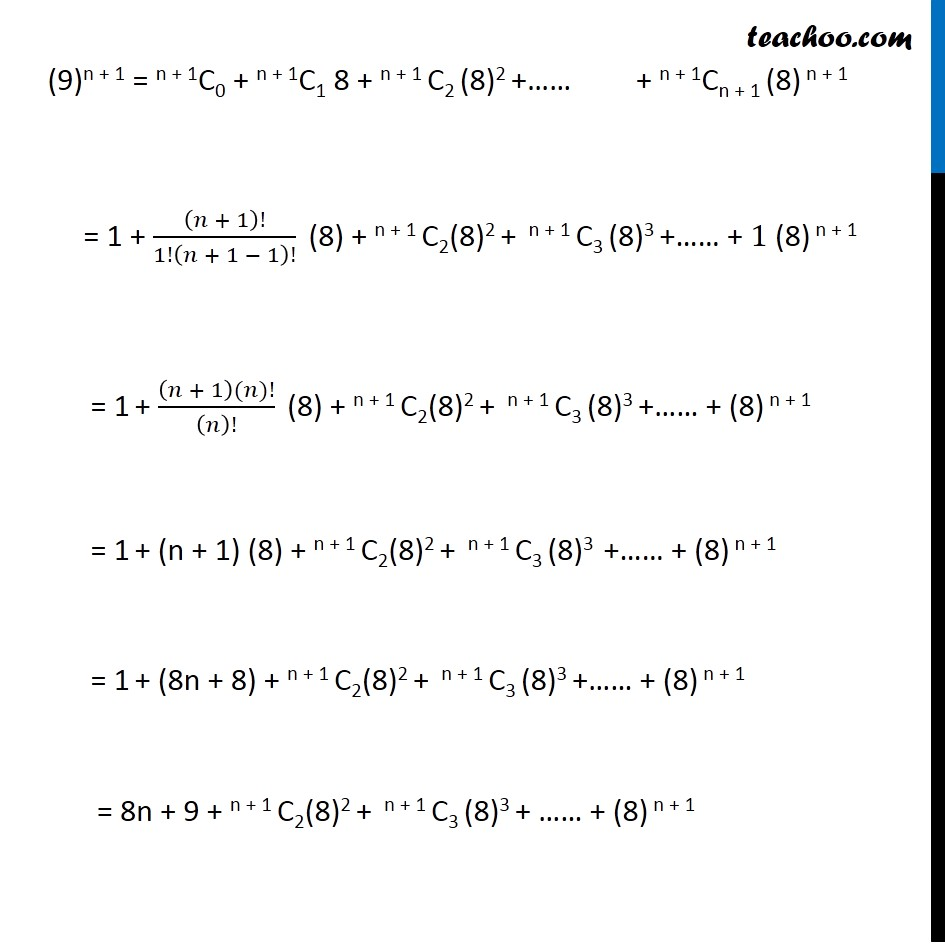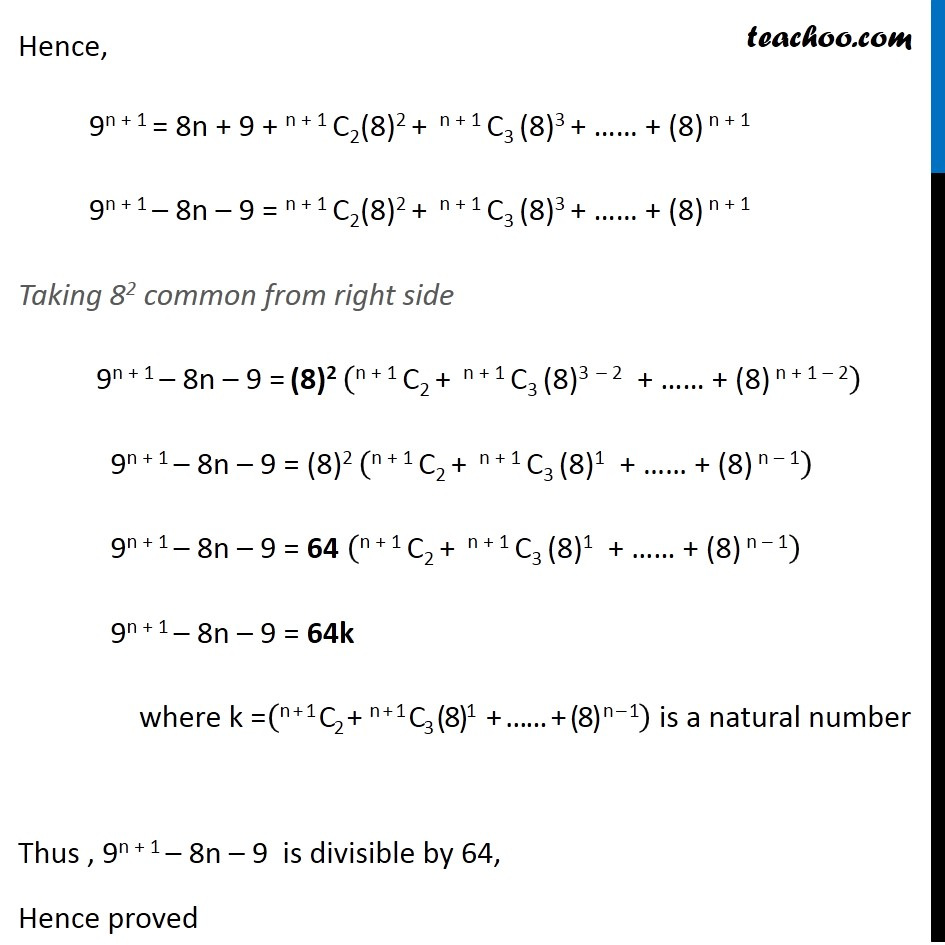Chapter 8 Class 11 Binomial Theorem

Class 11
Important Questions for exams Class 11Learn in your speed, with individual attention - Teachoo Maths 1-on-1 Class

### Transcript

Ex 7.1, 13 - Introduction Show that 9n+1 – 8n – 9 is divisible by 64, whenever n is a positive integer. Numbers divisible by 64 are 64 = 64 × 1 128 = 64 × 2 640 = 64 × 10 Any number divisible by 64 = 64 × Natural number Hence, In order to show that 9n+1 – 8n – 9 is divisible by 64, We have to prove that 9n+1 – 8n – 9 = 64k , where k is some natural number Ex 7.1, 13 Show that 9n+1 – 8n – 9 is divisible by 64, whenever n is a positive integer. In order to show that 9n+1 – 8n – 9 is divisible by 64, We have to prove that 9n+1 – 8n – 9 = 64k , where k is some natural number Writing (9)n+1 = (1 + 8) n+1 (9)n + 1 = n + 1C0 1(n + 1) + n + 1C1 1(n + 1) – 1 (8)1 + n + 1 C2 1n – 2(8)2 +…… + n + 1Cn + 1 (8) n + 1 We know that (a + b)n = nC0 an + nC1 an – 1 b 1 + nC2 an – 2 b2 + ….. + nCn bn Putting a = 1 ,b = 8 and n = n + 1, (9)n + 1 = n + 1C0 + n + 1C1 8 + n + 1 C2 (8)2 +…… + n + 1Cn + 1 (8) n + 1 = 1 + (𝑛 + 1)!/1!(𝑛 + 1 − 1)! (8) + n + 1 C2(8)2 + n + 1 C3 (8)3 +…… + 1 (8) n + 1 = 1 + ((𝑛 + 1)(𝑛)!)/(𝑛)! (8) + n + 1 C2(8)2 + n + 1 C3 (8)3 +…… + (8) n + 1 = 1 + (n + 1) (8) + n + 1 C2(8)2 + n + 1 C3 (8)3 +…… + (8) n + 1 = 1 + (8n + 8) + n + 1 C2(8)2 + n + 1 C3 (8)3 +…… + (8) n + 1 = 8n + 9 + n + 1 C2(8)2 + n + 1 C3 (8)3 + …… + (8) n + 1 Hence, 9n + 1 = 8n + 9 + n + 1 C2(8)2 + n + 1 C3 (8)3 + …… + (8) n + 1 9n + 1 – 8n – 9 = n + 1 C2(8)2 + n + 1 C3 (8)3 + …… + (8) n + 1 Taking 82 common from right side 9n + 1 – 8n – 9 = (8)2 ("n + 1 C2 + n + 1 C3 (8)3 – 2 + …… + (8) n + 1 – 2" ) 9n + 1 – 8n – 9 = (8)2 ("n + 1 C2 + n + 1 C3 (8)1 + …… + (8) n – 1" ) 9n + 1 – 8n – 9 = 64 ("n + 1 C2 + n + 1 C3 (8)1 + …… + (8) n – 1" ) 9n + 1 – 8n – 9 = 64k where k =("n + 1 C2 + n + 1 C3 (8)1 + …… + (8) n – 1" ) is a natural number Thus , 9n + 1 – 8n – 9 is divisible by 64, Hence proved